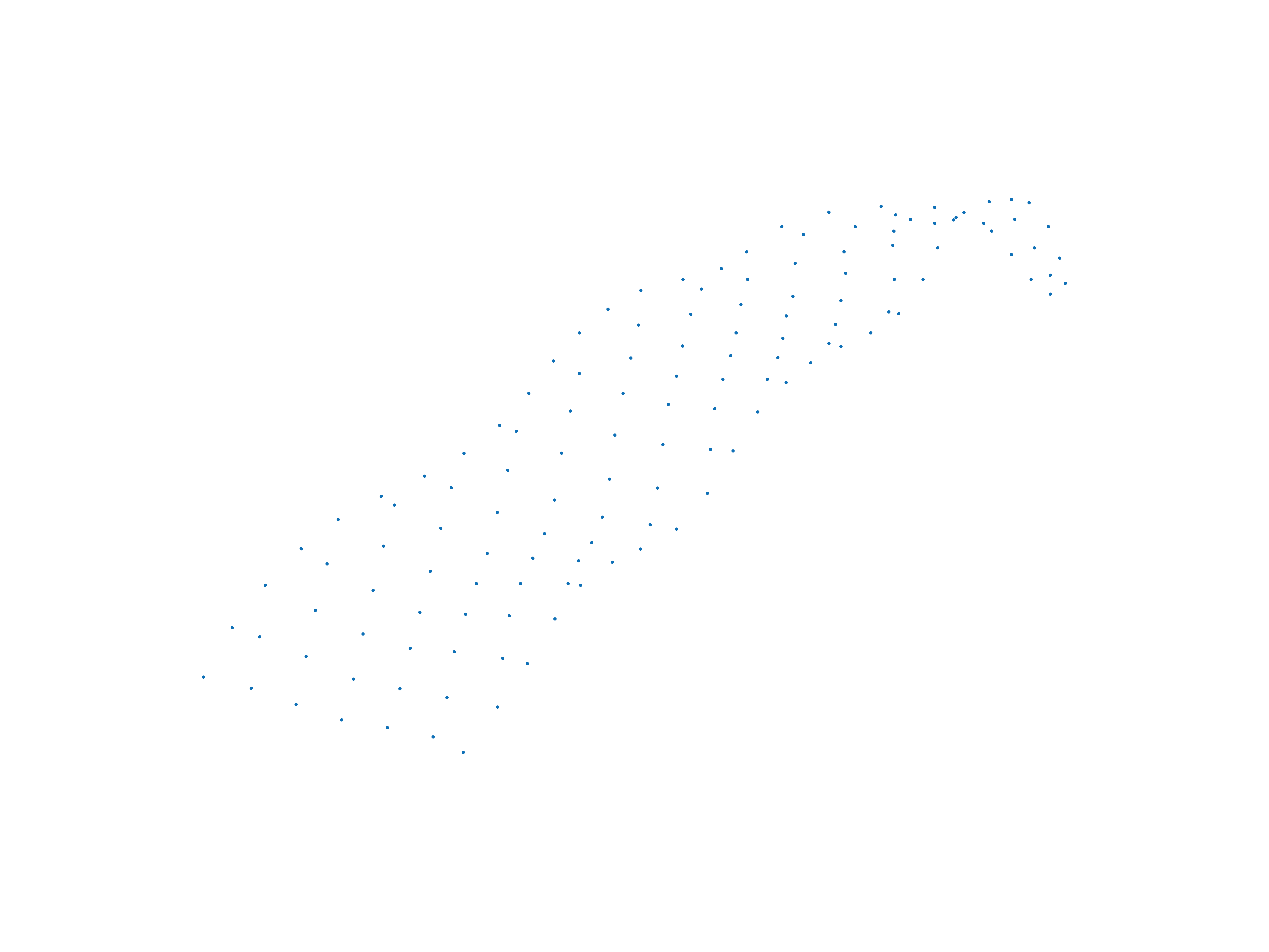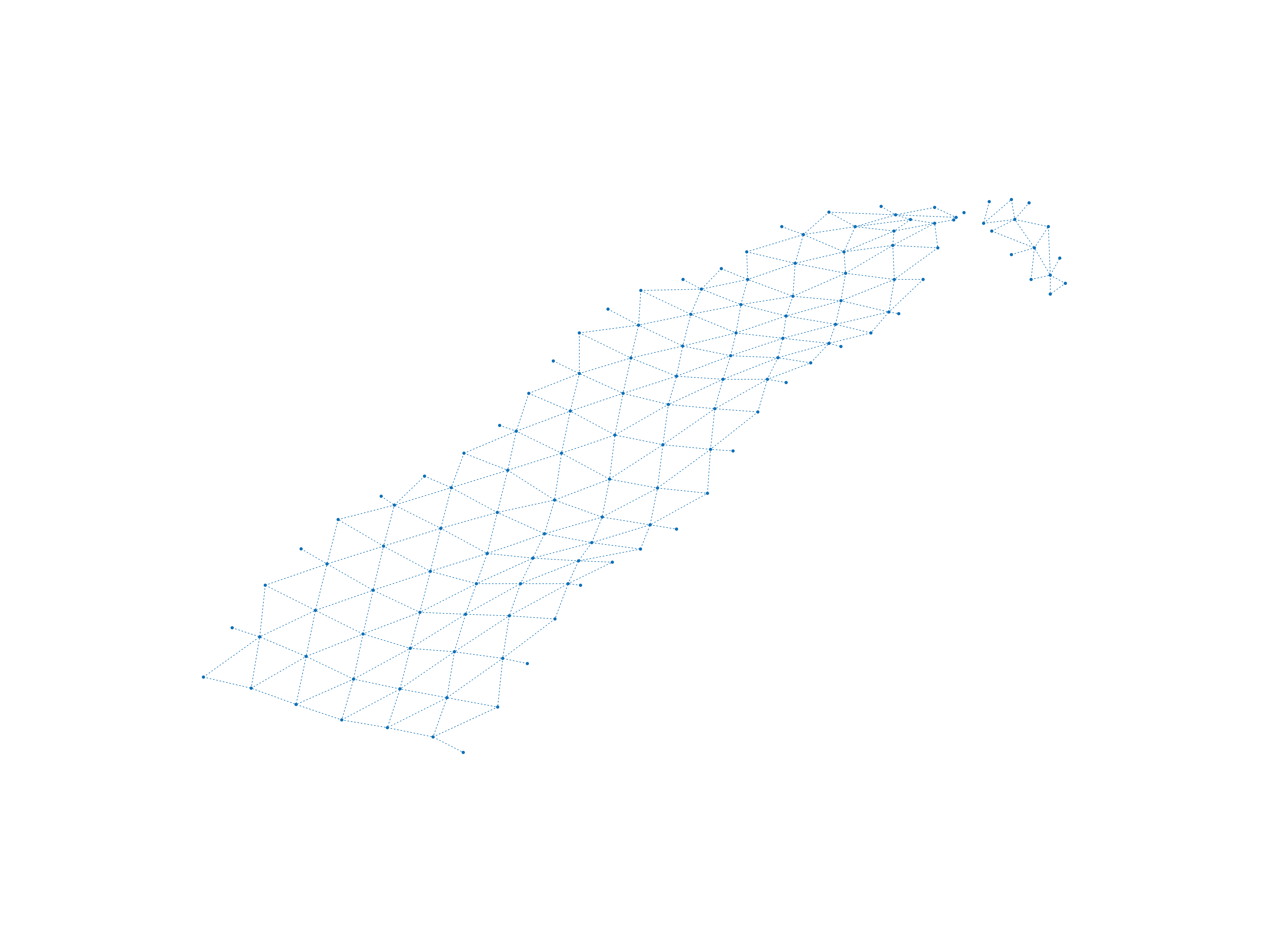Value Range: 0 – ∞
Unit: cm²

To measure the 3D leaf area of plants there are several steps involved. Starting from the 3D point cloud, all points that belong to the same sector are triangulated based on certain criteria. To create a triangle in the point cloud the points should be close to each other with no more point in between.The points in the point cloud do not have to be distributed equally in space, therefore the size of the triangles can vary. However, PlantEye can account for that by calculating the area of each individual triangle. Therefore, PlantEye can measure the real leaf area of inclined leaves other than 2D measurements that just take into account a projection of the leaf area.After the triangulation domains are searched within the plant, a domain is a group of triangles that forms a uniform surface. Each plant can have several domains. The number of domains is corresponding to the number of leaves; however, it is not an accurate leaf counting instrument. The 3D area of each domain is calculated by taking the sum of all included triangles. The total 3D leaf area of the plant is then calculated by taking the sum of the elementary triangles area.